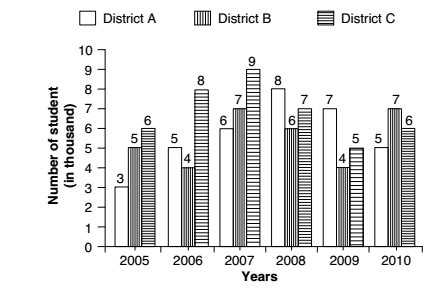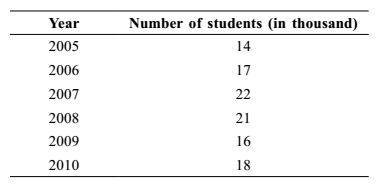## Bar graph

#### Data Interpretation

Direction: Study the following graph carefully to answer the questions that follow : Number of students (in thousand) enrolled in 3 different districts in 6 different years.1. What was the approximate average number of students enrolled in District A over all the years together?

1.  Required average = Sum of total number of students enrolled in District A in overall the years Total number of years

##### Correct Option: B

From above given Bar graph , we can see that
Number of students enrolled in District A in
years 2005 = 3000 students
years 2006 = 5000 students
years 2007 = 6000 students
years 2008 = 8000 students
years 2009 = 7000 students
years 2010 = 5000 students

 Required average = Sum of total number of students enrolled in District A in overall the years Total number of years

 Required average = 3000 + 5000 + 6000 + 8000 + 7000 + 5000 6

 Required average = 1000 x 34 = 5666 6

1. Total number of students enrolled in District A and District B together in the year 2010 was what percentage of the total number of students enrolled in District A in the year 2008?

1.  Required percentage = Total number of students enrolled in District A and District B in the year 2010 x 100% Total number of students enrolled in District A in the year 2008

##### Correct Option: A

Given in the graph :-
Number of students enrolled in District A in the year 2010 = 5000
Number of students enrolled in District B in the year 2010 = 7000
Total number of students enrolled in District A in the year 2008 = 8000

 Required percentage = Total number of students enrolled in District A and District B in the year 2010 x 100% Total number of students enrolled in District A in the year 2008

 Required percentage = ( 5000 + 7000 ) x 100 8000

 Required percentage = 12000 = 150 % 80

1. What was the percentage increase in enrollment in the number of students in District C in year 2007 as compared to that in the previous year?

1.  ∵ Required Percentage = Total change in enrollment x 100 Total number of students in District C in year 2006

##### Correct Option: D

As per given graph, We have
Number of students in District C in year 2007 = 9
Number of students in District C in year 2006 = 8
Total change in enrollment = Students in District C in year 2007 - Students in District Cin year 2006
Total change in enrollment = 9 - 8 = 1

 ∵ Required Percentage = Total change in enrollment x 100 Total number of students in District C in year 2006

 = 1 x 100 = 12.5 % 8

1. In which year was the number of students enrolled in all the three districts together the second highest?

1. Total number of students as per given, we can draw as below.##### Correct Option: C

From given graph , we can see that
Since , In 2008 year is the number of students enrolled in all the given 3 districts together the second highest.
Hence required Answer will be 2008 year .1. What was the difference between the number of students enrolled in all the 3 districts together in the year 2008 and the number of students enrolled in District B over all the years together?

1. Required Difference = Students in district B over all the years ( 2005 to 2010 ) − Students in 2008 in all 3 districts

##### Correct Option: A

Students enrolled in 2008 in all three districts ( A + B + C ) = 8000 + 6000 + 7000 = 21000
Students enrolled in district B over all the years ( 2005 to 2010 ) = 5000 + 4000 + 7000 + 6000 + 4000 + 7000 = 33000
∴ Required Difference = Students in district B over all the years ( 2005 to 2010 ) − Students in 2008 in all 3 districts
∴ Required Difference = 33000 − 21000 = 12000